# 60 dB Low Noise Audio Amplifier

Nov 05, 2020

The circuit below is a low noise low distortion 60 dB (Av=1000) gain block that can be used as an audio preamplifier for very low signal or noise measurements. A high performance LM4562NA dual op-amp is used. Each half of the op-amp uses a different circuit to demonstrate different usage. (The circuit could be easily duplicated in each half of the dual circuit for stereo application). The circuit is mounted in a well-shielded metal box and is powered by a +/- 6V battery pack providing the benefit of isolation from ground loops in line-powered supplies. A low voltage supply is within the supply range of many typical op-amps and is useful for lower level preamp applications such as noise measurements.

The first circuit (see schematic below, upper circuit) is a 60dB midband signal gain non-inverting amplifier with an f-3dB bandwidth of about 50 kHz. This is useful as a generic low noise, hi-gain block to boost low-level input signals Vin1 (down to about 1 μV) for further analysis (e.g. total noise measurements of circuits) or further processing.

The second circuit (schematic below, lower circuit) can be used in two different ways:
(1) as a unity gain inverting amplifier for measuring any op-amp's GBW product at nominal Vin2 signal levels (~ 1V input). In this case, the measured op-amp output voltage f-3dB rolloff frequency can be easily measured with good signal level, and for a typical op-amp open-loop rolloff gain characteristic, GBW ~ 1000*f-3dB.

(2) with no input signal (R14 shorted in schematic), to measure the en op-amp input noise voltage spectral density. The circuit is designed so that the en will dominate the output noise voltage for most op-amps tested. In this circuit, the "noise gain" (determined by feedback fraction from output to the NI input of op-amp) is 1000 within a bandwidth of 50 kHz (for the LM4562 op-amp). By measuring the total OUTPUT rms noise voltage Vout2 using an rms measuring voltmeter, the equivalent input opamp voltage noise spectral density, in V/√Hz is en ~ Vout2/1000/(1.57*f-3dB) where the 1.57*f-3dB is the total "noise bandwidth" assuming a single pole RC rolloff for the circuit.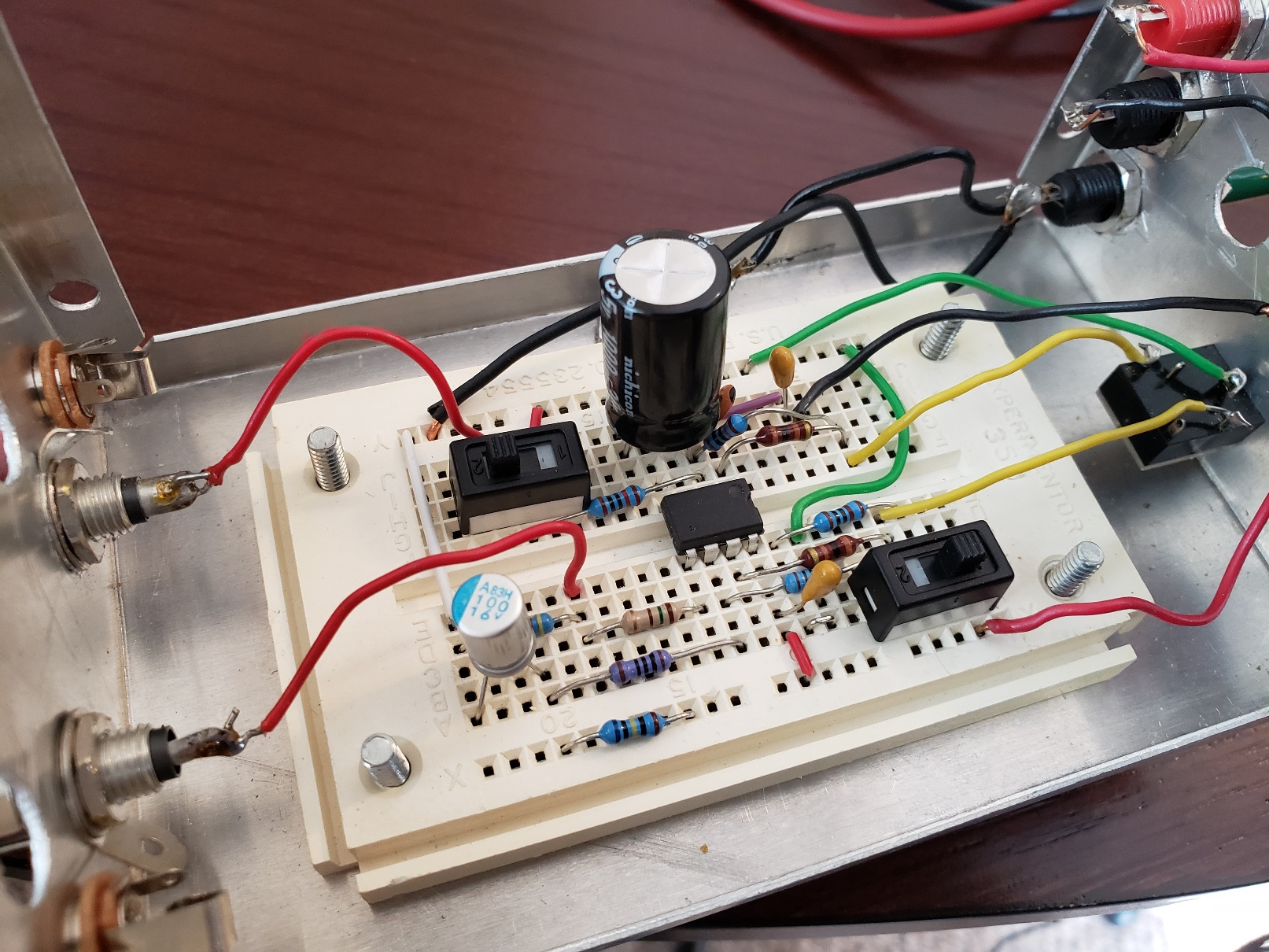## Bandwidth Measurements

Bode plots were performed for the two circuits above using a 10 MHz Analog Discovery dual channel oscilloscope/function generator along with an attenuator box. The setup is shown below. For the upper circuit (60dB signal gain in passband), the attenuator box was set at -60dB attenuation and the function generator signal was set at about 1V so input signal level at the 60dB amp was 1mV. For the lower circuit (0 dB signal gain in passband), there was no input signal attenuation since the gain in that circuit is unity.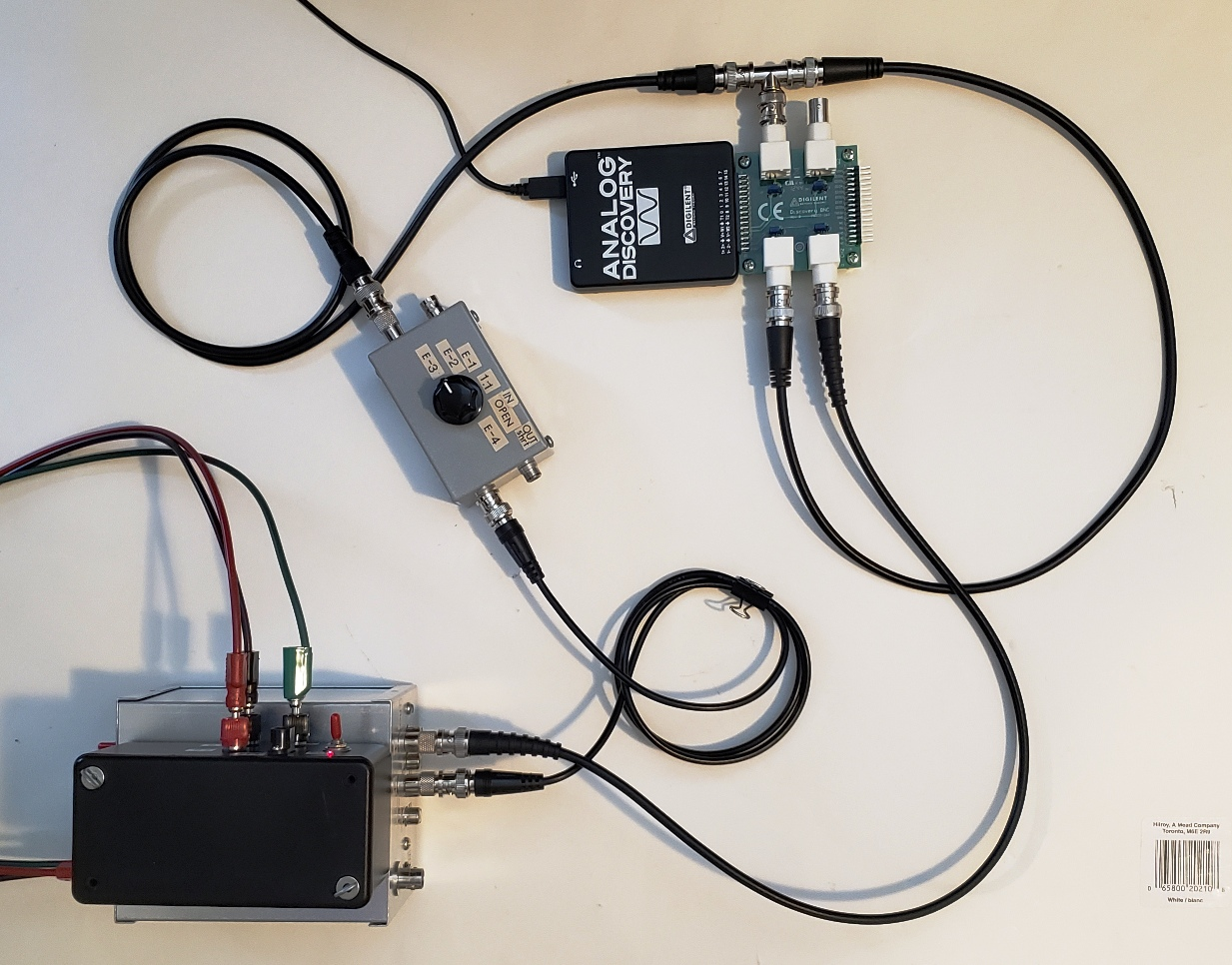The left plot below is for the noninverting 60dB signal gain circuit (Bode plot shows 0dB in passband because the output level is measured relative to the function generator signal BEFORE the attenuator box);
The right plot is for the inverting 0dB signal gain circuit. Note that the 16 Hz high pass filter in the feedback loop for this inverting circuit does NOT rolloff the signal gain (but does rolloff the noise gain).
Both circuits have almost identical f-3dB bandwidth of 50kHz and a single pole rolloff of -20dB/decade as expected: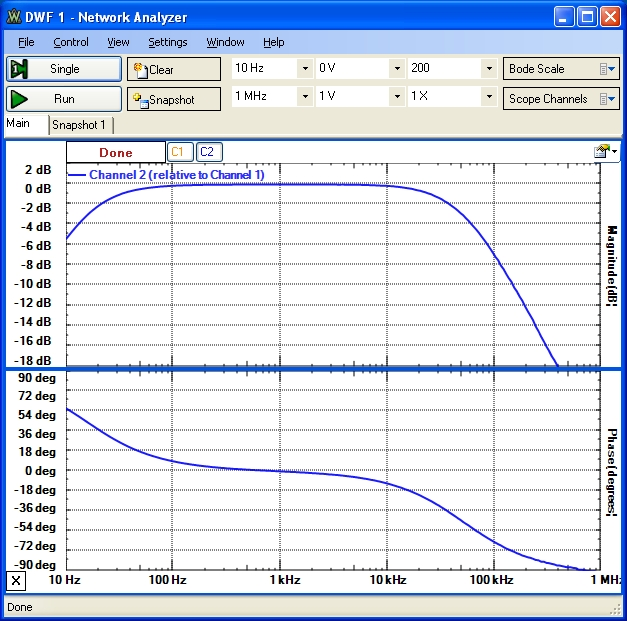## Example Noise Measurements: 46kHz, 20kHz, 20kHz A-Weighted

The following results are for the first circuit shown above with the input shorted (Rin=150 Ω) or open (Rin = 4.45 kΩ). Noise voltage measurements were performed using a high performance low noise Creative X-Fi Elite Pro sound card combined with a sampling application WaveMon measuring at a sampling rate of 96kHz (A high-sensitivity DMV capable of measuring true rms voltage down to the μV range could also be used). These measured rms noise output voltages Vout1 are then divided by 1000 (60dB) to obtain the equivalent input noise voltages Vi in the specified noise bandwidth. These are total rms noise measurements over the measurement bandwidth. Note that the total noise bandwidth will be determined by the combined rolloff of these tested 60dB amp's bandwidth of 50kHz (measured above) combined with the 46 kHz (fast) rolloff of the X-Fi Elite Pro sound card response. The 20 kHz 5th order noise filter used below has a very sharp rolloff which allows for a more standardized and relevant comparison of audio circuits discussed here. The A-Weight Filter and 5th order 20kHz low pass filter used here are described separately with the measured response shown below:

20kHz LP Filter at top; Combined 20kHz LPF and A-Weight Filter at bottom: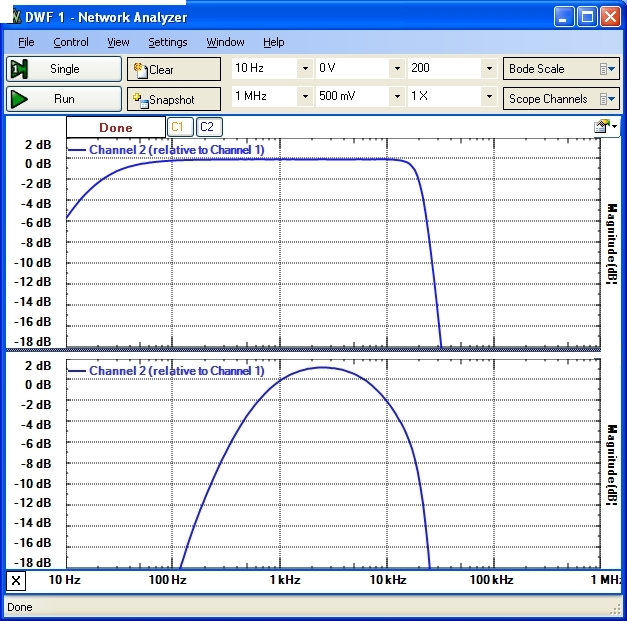The measured integrated noise results below illustrate the noise voltage differences expected for noise measurement bandwidths of 45-50 kHz, 20kHz and 20 kHz A-Weighted.
```
X-Fi Elite Pro Sound Card (46 kHz Bandwidth):  ~ 10μV noise floor
A-Weight Filter and 20 kHz 5th Order Filter:   ~ 10μV noise floor

Rin = 150 Ω  (noise dominated by op-amp en voltage noise)
60dB Amp 46kHz:   620μV   Vi=0.62μV
60dB Amp --> 20kHz LPF:  450μV   Vi=0.45μV
60dB Amp --> A-Weight Filter --> 20kHz LPF:  370μV   Vi=0.37μV

Rin = 4.45 kΩ (noise dominated by resistor thermal noise)
60dB Amp 46kHz:   2130μV    Vi=2.13μV
60dB Amp --> 20kHz LPF: 1600μV   Vi=1.6μV
60dB Amp --> A-Weight Filter --> 20kHz LPF:  1300μV   Vi=1.3μV
```

The measured results for this 60dB LM4562NA (GBW=50MHz; en=3nV/√Hz; in=2pA/√Hz) high-gain amplifier circuit are in good agreement with the total integrated output voltage noise expected from detailed noise transfer function modelling predictions for this circuit/op-amp and demonstrate a design that can be used for analog signal and noise preamplification for measurement down to about 0.5μVrms. The input resistance of the circuit is 4.3 kΩ which is fairly low. For noise measurements of devices connected to the input, this may be too low and may load the circuit being measured down. Rp should be raised so that it is 100x or greater than the output impedance of the connected input device to be measured or amplified.

## 60 dB Low Noise Preamp: Single op-amps

The example above used a double op-amp layout. The example below shows the same circuit design (for the 1st part of the op-amp above). The single op-amp used can be easily switched to compare noise performance of different op-amps, or as a general purpose low noise single gain block. Duplication of the basic circuit with a second identical op-amp is easy for stereo applications: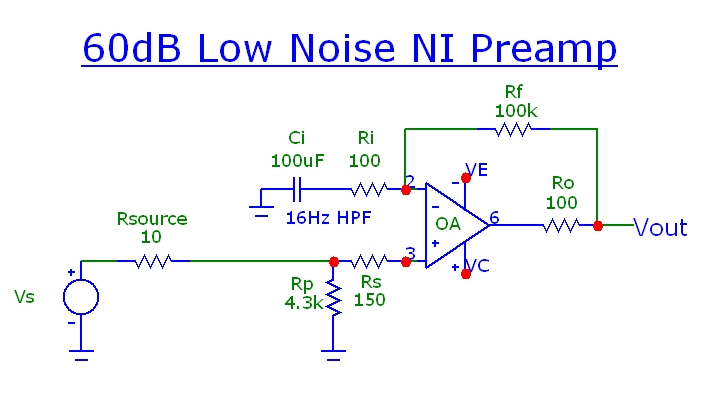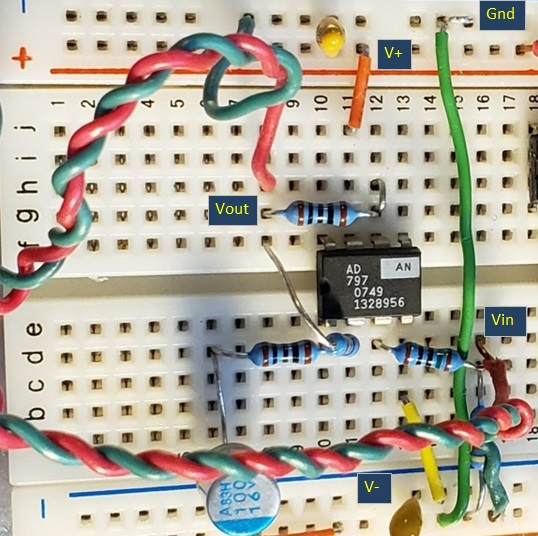The measured frequency response for a precision LT1028 op-amp (GBW=75 MHz; en=1nV/√Hz; in=1pA/√Hz) is shown for (1) no compensation capacitance (solid) and (2) 100pF compensation capacitor between pins 5 and 6 (dotted):